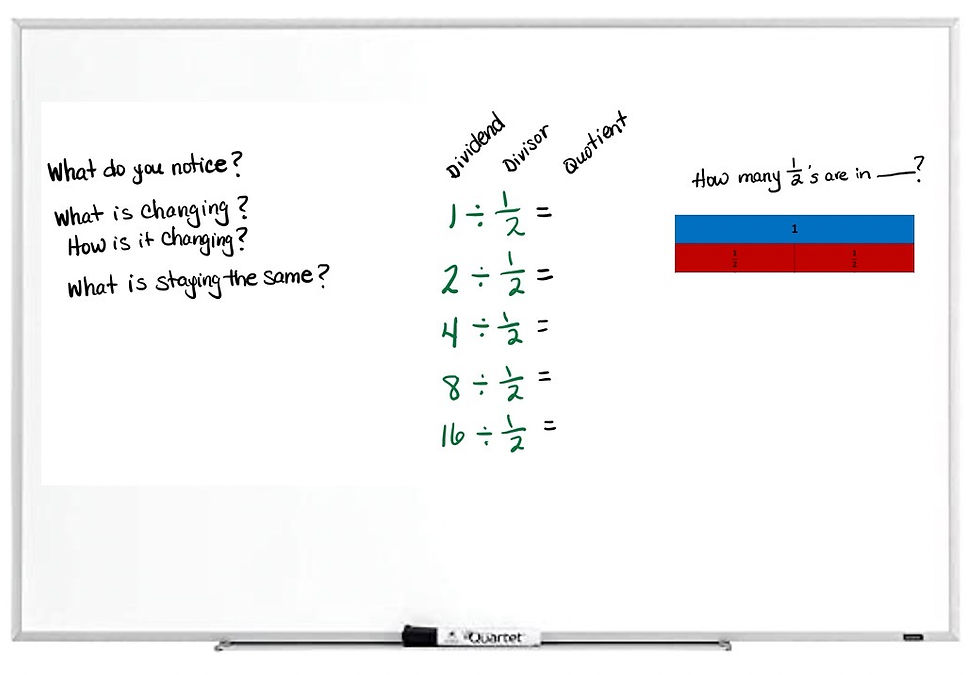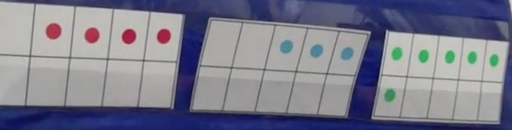top of page
Search

# Number Strings: A Different Kind of Number Talk

When I first started using Number Talks in the classroom, I would randomly choose problems for students to solve. I would ask them to think of different strategies for solving the problem, discuss with a partner, and then I would record the strategies on the whiteboard to highlight student thinking. (See our blog post, How Can We Adapt Number Talks for Distance Learning? to learn more about Number Talks).

While these Number Talks were helping students make progress solving problems and communicating their thinking, there were still those who weren’t using efficient methods or strategies based on place value or properties of operations. This dilemma led me to learn about Number Strings.

Math educator Cathy Fosnot describes a Number String “as a set of related math problems, crafted to support students to construct big ideas about mathematics and build their own strategies” (Fosnot & Dolk, 2008).

### A Division of Fractions Number StringIn the following video clip, you’ll see Andrea Barraugh teaching the Number String shown above. The first problem serves as a scaffold for the subsequent problems, and the string is purposely crafted to help students see patterns and make predictions about the quotients.

When watching the video clip, notice how Andrea introduces the first problem by helping students interpret 1 divided by ½ as, “How many halves are in one whole?” She provides a sentence frame for students to use when thinking about the other problems in the string:

How many _____ are in ______?

Andrea also models the problems using fraction strips to help students “see” what the quantities look like.

### A Number String for First and Second Graders

Take a look at the following Number String that I shared with a group of second graders. I started with something they were all familiar with, 10 + 10. Starting with easy problems in a Number String gives students confidence and a familiar starting place to solve subsequent problems. After posing 10+10, I showed the class 10+11, then 12+13, and finally 20+11:Knowing that 10+10 is 20, most students solved 10+11 by using 10+10 and then adding the one to get to 21. The purpose in this short string was to encourage students to use their knowledge of place value, rather than counting on by ones from the 10. To solve 12+13, some students started with 12+10, then added the 3. Others immediately saw 2+3 makes 5, then added the two 10s. When we got to 20+11, most students started with 20+10 and then added the 1. I was surprised and delighted that this string of related problems seemed to pave a pathway for using efficient methods that reinforced place value.

Here’s a Number String crafted by Cathy Fosnot that encourages students to make use of the pattern of adding a 10. Notice how the first problem is the scaffold for the second:### Encouraging Doubles Plus One

If my focus were to encourage students to use doubles plus one, my string might look like this:(12+12 is 24; and 24+1 is 25 or, 13+13 is 26; and 26-1 is 25)

### Encouraging Subtracting Ten

The following Number String encourages students to keep the first number whole and then subtract 10s:The beauty of a Number String is that students can still solve the problems in ways that make sense to them. But because the problems are intentionally related, students begin to catch on to the pattern and start to practice important skills and concepts.

### Number Strings for Grades TK, K, and 1

Number Strings don’t have to consist of a string of problems. You can pose one problem that consists of a string of numbers, or in the following case, a string of ten-frames. This ten-frame string might be used to encourage students to find a 10 and then add on from there.Here’s another Number String that encourages making a 10. Notice that the problems don’t necessarily build on one another, but each string includes addends that will make a 10:### A Multiplication Number String

The following multiplication string has the power to encourage students to notice patterns and then use strategies such as doubling and big ideas such as the distributive property to solve problems.

2x7 (easy fact to begin with) 4x7 (double 2x7 to get to 4x7) 3x7 (2x7 plus one more group of 7 or, 4x7 subtract one group of 7) 7x7 (4x7 + 3x7 to get to 7x7)

This string encourages students to break numbers apart to create easier problems. For example, the problem 7x7 can be thought of as 4 groups of 7 plus 3 groups of 7. As Mark Alcorn reminds us,

“Promoting efficient strategies with basic multiplication facts is the important conceptual knowledge that can be developed and applied later in more complex mathematics content. An example of a concept that builds through arithmetic into algebra is the distributive property (Alcorn, 2008).”

### The Power of Number Strings

While we want to honor the ways in which students naturally think about and solve problems, there are benefits to strategically and intentionally crafting Number Strings so that students can develop big ideas (place value, properties of operations) by noticing patterns, building on what they know, and then sharing these ideas with other students.

For more Number String ideas, see Minilessons for Extending Addition and Subtraction (Fosnot & Dolk,2008).